# Thermo chapter 1

24. Feb 2014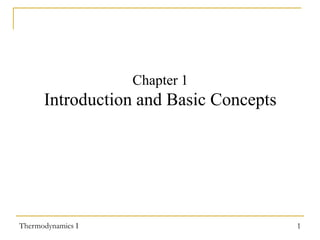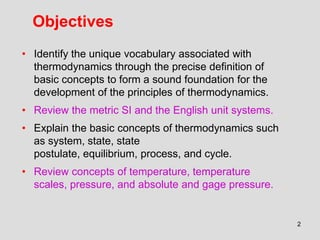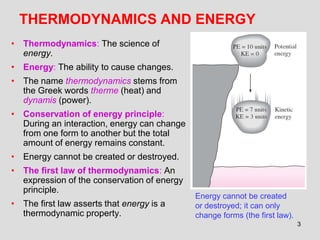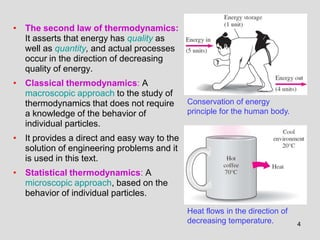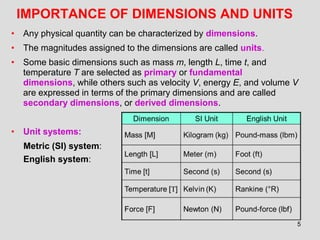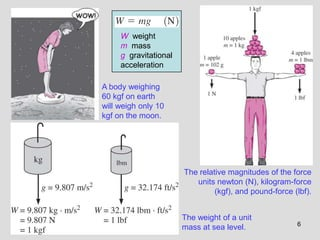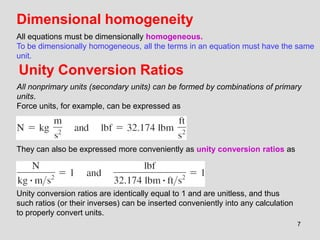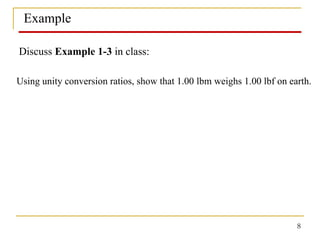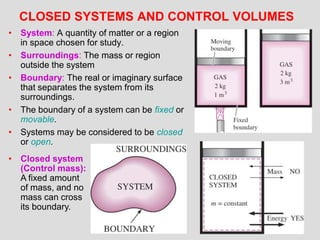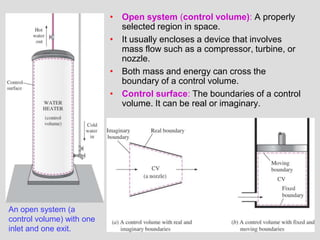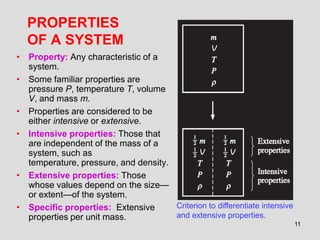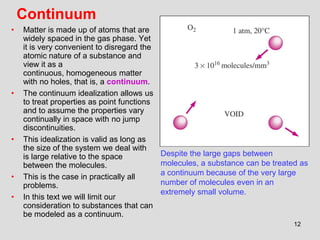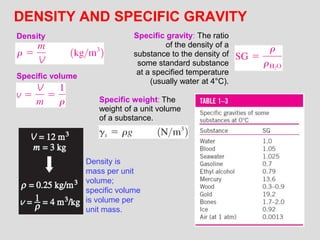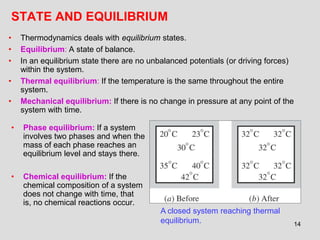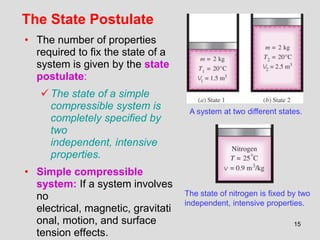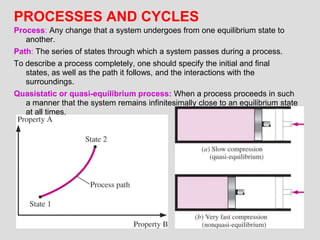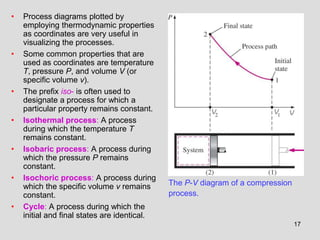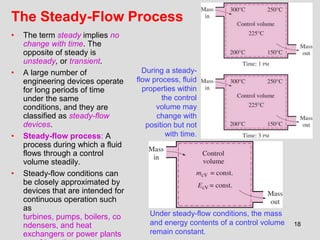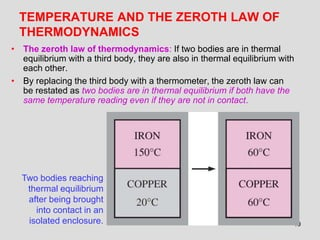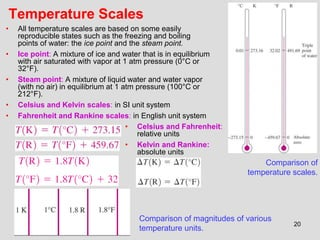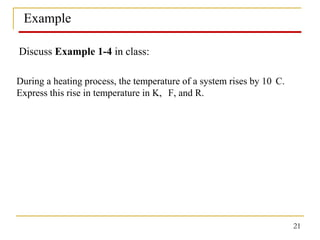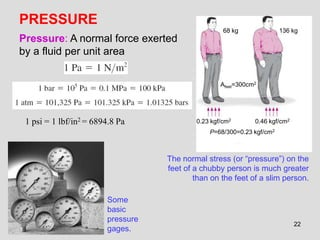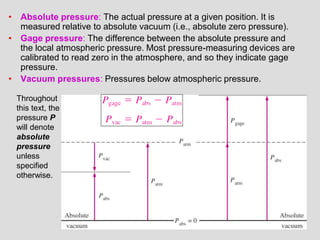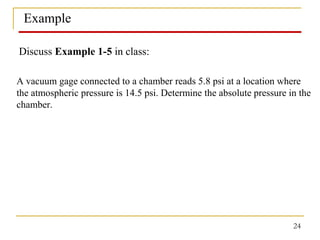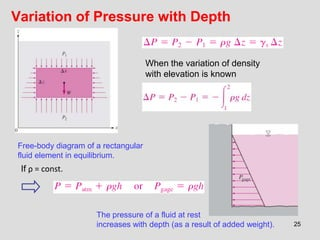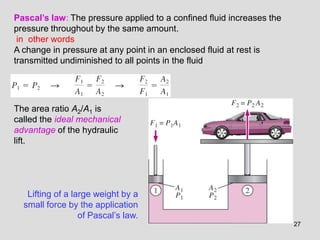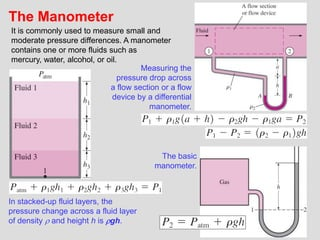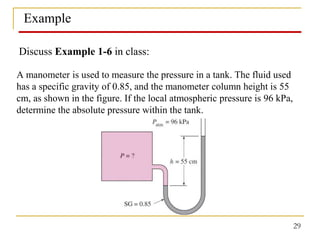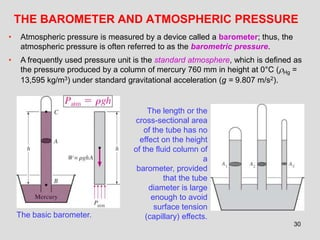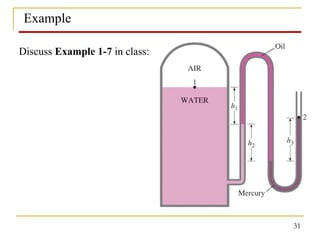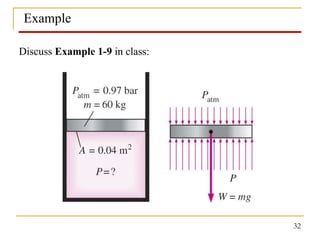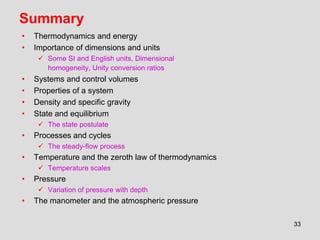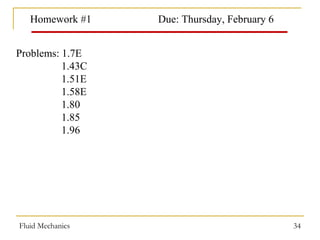1 von 34

### Thermo chapter 1

• 1. Chapter 1 Introduction and Basic Concepts Thermodynamics I 1
• 2. Objectives • Identify the unique vocabulary associated with thermodynamics through the precise definition of basic concepts to form a sound foundation for the development of the principles of thermodynamics. • Review the metric SI and the English unit systems. • Explain the basic concepts of thermodynamics such as system, state, state postulate, equilibrium, process, and cycle. • Review concepts of temperature, temperature scales, pressure, and absolute and gage pressure. 2
• 3. THERMODYNAMICS AND ENERGY • Thermodynamics: The science of energy. • Energy: The ability to cause changes. • The name thermodynamics stems from the Greek words therme (heat) and dynamis (power). • Conservation of energy principle: During an interaction, energy can change from one form to another but the total amount of energy remains constant. • Energy cannot be created or destroyed. • The first law of thermodynamics: An expression of the conservation of energy principle. • The first law asserts that energy is a thermodynamic property. Energy cannot be created or destroyed; it can only change forms (the first law). 3
• 4. • The second law of thermodynamics: It asserts that energy has quality as well as quantity, and actual processes occur in the direction of decreasing quality of energy. • Classical thermodynamics: A macroscopic approach to the study of thermodynamics that does not require a knowledge of the behavior of individual particles. Conservation of energy principle for the human body. • It provides a direct and easy way to the solution of engineering problems and it is used in this text. • Statistical thermodynamics: A microscopic approach, based on the behavior of individual particles. Heat flows in the direction of decreasing temperature. 4
• 5. IMPORTANCE OF DIMENSIONS AND UNITS • Any physical quantity can be characterized by dimensions. • The magnitudes assigned to the dimensions are called units. • Some basic dimensions such as mass m, length L, time t, and temperature T are selected as primary or fundamental dimensions, while others such as velocity V, energy E, and volume V are expressed in terms of the primary dimensions and are called secondary dimensions, or derived dimensions. • Unit systems: Metric (SI) system: English system: 5
• 6. W weight m mass g gravitational acceleration A body weighing 60 kgf on earth will weigh only 10 kgf on the moon. The relative magnitudes of the force units newton (N), kilogram-force (kgf), and pound-force (lbf). The weight of a unit mass at sea level. 6
• 7. Dimensional homogeneity All equations must be dimensionally homogeneous. To be dimensionally homogeneous, all the terms in an equation must have the same unit. Unity Conversion Ratios All nonprimary units (secondary units) can be formed by combinations of primary units. Force units, for example, can be expressed as They can also be expressed more conveniently as unity conversion ratios as Unity conversion ratios are identically equal to 1 and are unitless, and thus such ratios (or their inverses) can be inserted conveniently into any calculation to properly convert units. 7
• 8. Example Discuss Example 1-3 in class: Using unity conversion ratios, show that 1.00 lbm weighs 1.00 lbf on earth. 8
• 9. CLOSED SYSTEMS AND CONTROL VOLUMES • System: A quantity of matter or a region in space chosen for study. • Surroundings: The mass or region outside the system • Boundary: The real or imaginary surface that separates the system from its surroundings. • The boundary of a system can be fixed or movable. • Systems may be considered to be closed or open. • Closed system (Control mass): A fixed amount of mass, and no mass can cross its boundary. 9
• 10. • Open system (control volume): A properly selected region in space. • It usually encloses a device that involves mass flow such as a compressor, turbine, or nozzle. • Both mass and energy can cross the boundary of a control volume. • Control surface: The boundaries of a control volume. It can be real or imaginary. An open system (a control volume) with one inlet and one exit. 10
• 11. PROPERTIES OF A SYSTEM • Property: Any characteristic of a system. • Some familiar properties are pressure P, temperature T, volume V, and mass m. • Properties are considered to be either intensive or extensive. • Intensive properties: Those that are independent of the mass of a system, such as temperature, pressure, and density. • Extensive properties: Those whose values depend on the size— or extent—of the system. Criterion to differentiate intensive • Specific properties: Extensive and extensive properties. properties per unit mass. 11
• 12. Continuum • • • • • Matter is made up of atoms that are widely spaced in the gas phase. Yet it is very convenient to disregard the atomic nature of a substance and view it as a continuous, homogeneous matter with no holes, that is, a continuum. The continuum idealization allows us to treat properties as point functions and to assume the properties vary continually in space with no jump discontinuities. This idealization is valid as long as the size of the system we deal with is large relative to the space between the molecules. This is the case in practically all problems. In this text we will limit our consideration to substances that can be modeled as a continuum. Despite the large gaps between molecules, a substance can be treated as a continuum because of the very large number of molecules even in an extremely small volume. 12
• 13. DENSITY AND SPECIFIC GRAVITY Density Specific volume Specific gravity: The ratio of the density of a substance to the density of some standard substance at a specified temperature (usually water at 4°C). Specific weight: The weight of a unit volume of a substance. Density is mass per unit volume; specific volume is volume per unit mass. 13
• 14. STATE AND EQUILIBRIUM • • • • • Thermodynamics deals with equilibrium states. Equilibrium: A state of balance. In an equilibrium state there are no unbalanced potentials (or driving forces) within the system. Thermal equilibrium: If the temperature is the same throughout the entire system. Mechanical equilibrium: If there is no change in pressure at any point of the system with time. • Phase equilibrium: If a system involves two phases and when the mass of each phase reaches an equilibrium level and stays there. • Chemical equilibrium: If the chemical composition of a system does not change with time, that is, no chemical reactions occur. A closed system reaching thermal equilibrium. 14
• 15. The State Postulate • The number of properties required to fix the state of a system is given by the state postulate:  The state of a simple compressible system is completely specified by two independent, intensive properties. • Simple compressible system: If a system involves no electrical, magnetic, gravitati onal, motion, and surface tension effects. A system at two different states. The state of nitrogen is fixed by two independent, intensive properties. 15
• 16. PROCESSES AND CYCLES Process: Any change that a system undergoes from one equilibrium state to another. Path: The series of states through which a system passes during a process. To describe a process completely, one should specify the initial and final states, as well as the path it follows, and the interactions with the surroundings. Quasistatic or quasi-equilibrium process: When a process proceeds in such a manner that the system remains infinitesimally close to an equilibrium state at all times. 16
• 17. • • • • • • • Process diagrams plotted by employing thermodynamic properties as coordinates are very useful in visualizing the processes. Some common properties that are used as coordinates are temperature T, pressure P, and volume V (or specific volume v). The prefix iso- is often used to designate a process for which a particular property remains constant. Isothermal process: A process during which the temperature T remains constant. Isobaric process: A process during which the pressure P remains constant. Isochoric process: A process during which the specific volume v remains constant. Cycle: A process during which the initial and final states are identical. The P-V diagram of a compression process. 17
• 18. The Steady-Flow Process • • • • The term steady implies no change with time. The opposite of steady is unsteady, or transient. A large number of engineering devices operate for long periods of time under the same conditions, and they are classified as steady-flow devices. Steady-flow process: A process during which a fluid flows through a control volume steadily. Steady-flow conditions can be closely approximated by devices that are intended for continuous operation such as turbines, pumps, boilers, co ndensers, and heat exchangers or power plants During a steadyflow process, fluid properties within the control volume may change with position but not with time. Under steady-flow conditions, the mass and energy contents of a control volume remain constant. 18
• 19. TEMPERATURE AND THE ZEROTH LAW OF THERMODYNAMICS • The zeroth law of thermodynamics: If two bodies are in thermal equilibrium with a third body, they are also in thermal equilibrium with each other. • By replacing the third body with a thermometer, the zeroth law can be restated as two bodies are in thermal equilibrium if both have the same temperature reading even if they are not in contact. Two bodies reaching thermal equilibrium after being brought into contact in an isolated enclosure. 19
• 20. Temperature Scales • • • • • All temperature scales are based on some easily reproducible states such as the freezing and boiling points of water: the ice point and the steam point. Ice point: A mixture of ice and water that is in equilibrium with air saturated with vapor at 1 atm pressure (0°C or 32°F). Steam point: A mixture of liquid water and water vapor (with no air) in equilibrium at 1 atm pressure (100°C or 212°F). Celsius and Kelvin scales: in SI unit system Fahrenheit and Rankine scales: in English unit system • Celsius and Fahrenheit: relative units • Kelvin and Rankine: absolute units Comparison of temperature scales. Comparison of magnitudes of various temperature units. 20
• 21. Example Discuss Example 1-4 in class: During a heating process, the temperature of a system rises by 10 C. Express this rise in temperature in K, F, and R. 21
• 22. PRESSURE 68 kg 136 kg Pressure: A normal force exerted by a fluid per unit area Afeet=300cm2 1 psi = 1 lbf/in2 = 6894.8 Pa 0.23 kgf/cm2 0.46 kgf/cm2 P=68/300=0.23 kgf/cm2 The normal stress (or “pressure”) on the feet of a chubby person is much greater than on the feet of a slim person. Some basic pressure gages. 22
• 23. • Absolute pressure: The actual pressure at a given position. It is measured relative to absolute vacuum (i.e., absolute zero pressure). • Gage pressure: The difference between the absolute pressure and the local atmospheric pressure. Most pressure-measuring devices are calibrated to read zero in the atmosphere, and so they indicate gage pressure. • Vacuum pressures: Pressures below atmospheric pressure. Throughout this text, the pressure P will denote absolute pressure unless specified otherwise. 23
• 24. Example Discuss Example 1-5 in class: A vacuum gage connected to a chamber reads 5.8 psi at a location where the atmospheric pressure is 14.5 psi. Determine the absolute pressure in the chamber. 24
• 25. Variation of Pressure with Depth When the variation of density with elevation is known Free-body diagram of a rectangular fluid element in equilibrium. If ρ = const. The pressure of a fluid at rest increases with depth (as a result of added weight). 25
• 26. In a room filled with a gas, the variation of pressure with height is negligible. Pressure in a liquid at rest increases linearly with distance from the free surface. The pressure is the same at all points on a horizontal plane in a given fluid regardless of geometry, provided that the points are interconnected by the same fluid. 26
• 27. Pascal’s law: The pressure applied to a confined fluid increases the pressure throughout by the same amount. in other words A change in pressure at any point in an enclosed fluid at rest is transmitted undiminished to all points in the fluid The area ratio A2/A1 is called the ideal mechanical advantage of the hydraulic lift. Lifting of a large weight by a small force by the application of Pascal’s law. 27
• 28. The Manometer It is commonly used to measure small and moderate pressure differences. A manometer contains one or more fluids such as mercury, water, alcohol, or oil. Measuring the pressure drop across a flow section or a flow device by a differential manometer. The basic manometer. In stacked-up fluid layers, the pressure change across a fluid layer of density  and height h is gh. 28
• 29. Example Discuss Example 1-6 in class: A manometer is used to measure the pressure in a tank. The fluid used has a specific gravity of 0.85, and the manometer column height is 55 cm, as shown in the figure. If the local atmospheric pressure is 96 kPa, determine the absolute pressure within the tank. 29
• 30. THE BAROMETER AND ATMOSPHERIC PRESSURE • Atmospheric pressure is measured by a device called a barometer; thus, the atmospheric pressure is often referred to as the barometric pressure. • A frequently used pressure unit is the standard atmosphere, which is defined as the pressure produced by a column of mercury 760 mm in height at 0°C (Hg = 13,595 kg/m3) under standard gravitational acceleration (g = 9.807 m/s2). The basic barometer. The length or the cross-sectional area of the tube has no effect on the height of the fluid column of a barometer, provided that the tube diameter is large enough to avoid surface tension (capillary) effects. 30
• 33. Summary • • Thermodynamics and energy Importance of dimensions and units  Some SI and English units, Dimensional homogeneity, Unity conversion ratios • • • • Systems and control volumes Properties of a system Density and specific gravity State and equilibrium  The state postulate • Processes and cycles  The steady-flow process • Temperature and the zeroth law of thermodynamics  Temperature scales • Pressure  Variation of pressure with depth • The manometer and the atmospheric pressure 33
• 34. Homework #1 Due: Thursday, February 6 Problems: 1.7E 1.43C 1.51E 1.58E 1.80 1.85 1.96 Fluid Mechanics 34